• Call Now

1800-102-2727•

# Modulation

Suresh wants to talk to Hari, who is 3 km far from him. Then how will they communicate? Yes, they need a certain device like mobile or telephone. Since Suresh’s voice will be sent to Hari in the form of audio signals which are very weak, it has to be sent with a strong signal which will act as a carrier in this case. This is similar to an example that Suresh wants to go to meet Hari at 3 km distance but this distance is quite far if Suresh wants to go by walk. So Suresh will take a ride on his bike and reach Hari. Hence, for this case Suresh is the weak signal and bike is the carrier signal. The process is called modulation.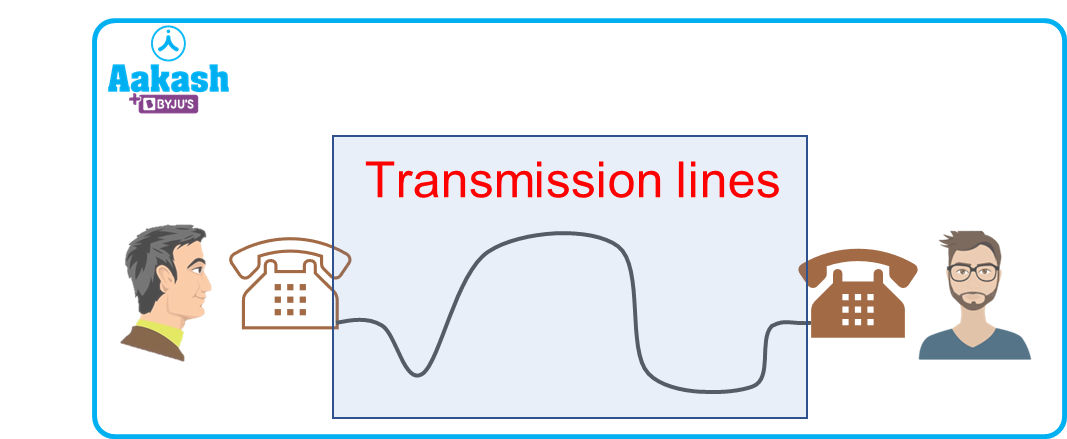Table of content

• What is Modulation?
• Why do we need modulation?
• Types of modulation
• Practice problem
• FAQs

## What is Modulation?

Modulation is the process of providing sufficient strength to a message signal (information signal) by superposition of a high-frequency signal called a carrier signal. Since the message signal is weak in form to travel a long distance, a carrier signal is to be used which takes the message signal from transmitter to receiver.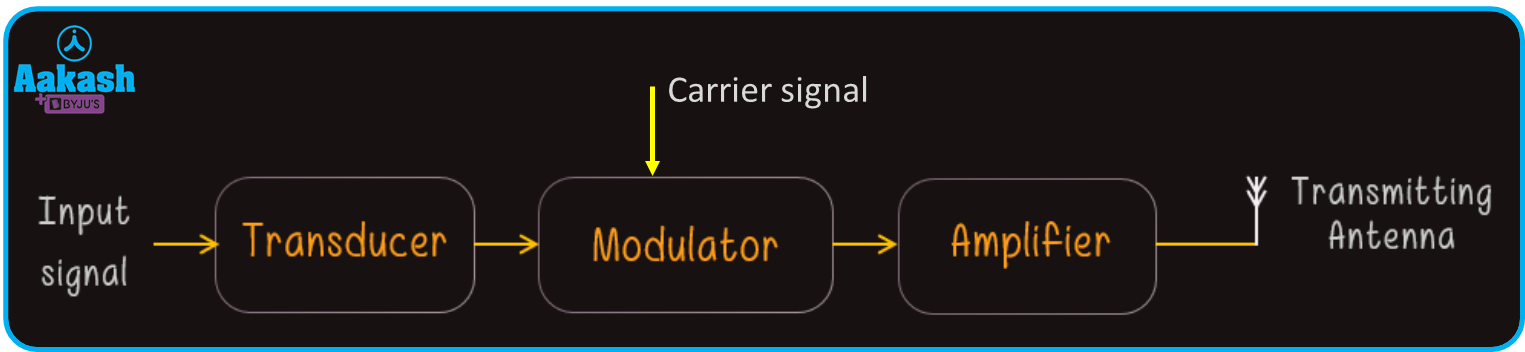Fig shows the modulation process of a input signal

Above figure shows the basic structure of modulation. Message signal is also known as the baseband signal. The modulation is done with the help of a device called a modulator.

## Why do we need modulation?

In this digitally progressive era, the modulation process plays a key role in the transmission of signals in less time to a longer distance. The signals like audio signals which are having frequencies between 20 Hz to 20 kHz can not be directly transmitted for a long distance.This is due to the low energy of the wave. There are some key points explained below that describe the need for modulation.

1. Size of antenna:

Let’s suppose, a signal having 10 kHz frequency is to be transmitted to a receiver, then the corresponding wavelength is given by,

$\lambda =\frac{c}{f}$

where, c= Speed of wave and f= audio signal frequency.

Then, the wavelength of the signal will be,

Now we know the minimum length of an antenna should be /4. Then,

Hence the height of the antenna for the given frequency is 7500 m which is practically impossible to construct.

Now, here we can use the modulation method by using a high frequency carrier wave to transmit the message signal so that the height of the antenna can be reduced.

2. Effective power radiated by the antenna:

As high power is needed for a good transmission, wavelength () should be small and for that, frequency should be high. Power of the signal is given by,

Where, l= Length of the antenna

For the same value of l, higher the wavelength, the lower the power transmitted.

3. Mixing of signals from other transmitters:

There are a lot of signals which are transmitted and can get mixed up if the signals are not distinguished from each other. This is done by dividing the signals into bands at high frequencies.

## Types of modulation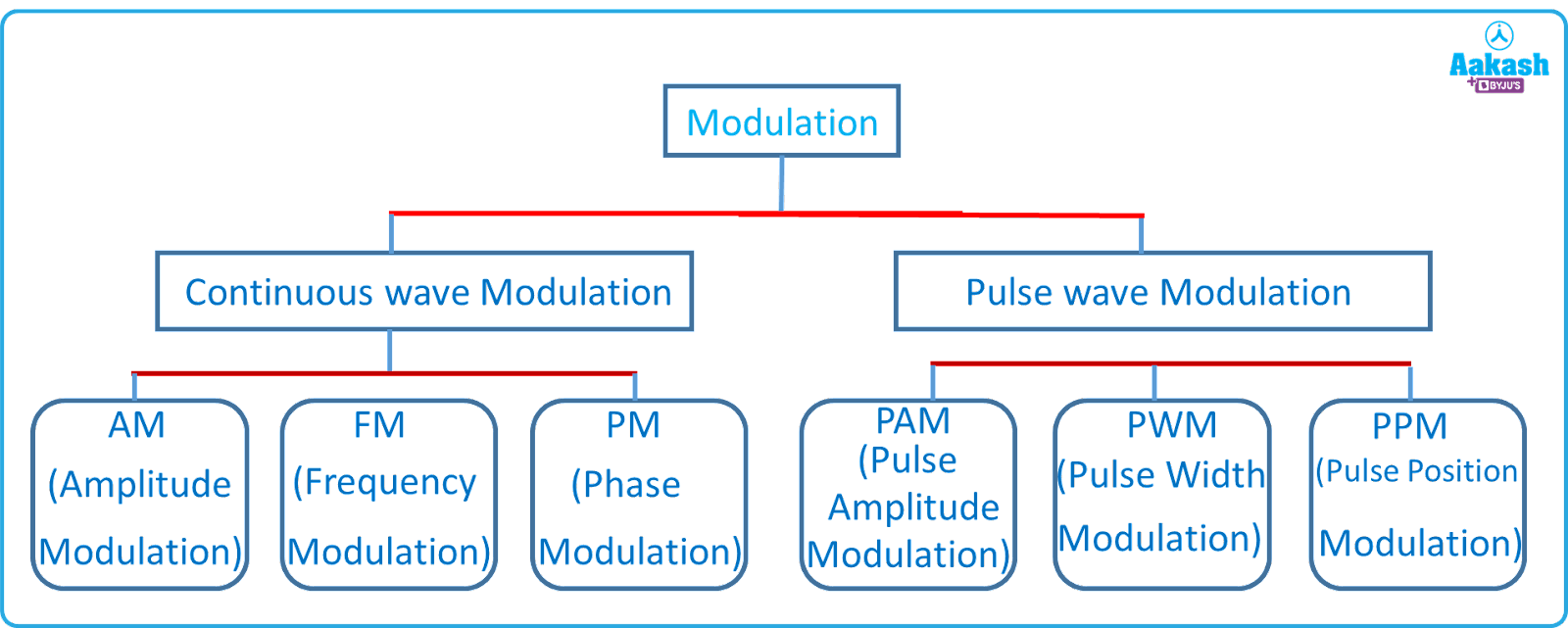There are two types of modulation based on the type of carrier wave:

1. Continuous wave modulation
2. Pulse wave modulation

A continuous sinusoidal carrier signal is expressed as;

(a) Continuous wave modulation: Any one of three characteristics (V0, , ) can be varied in accordance with modulating baseband (AF) signal, giving rise to;

1. Amplitude modulation
2. Frequency modulation
3. Phase modulation
1. Amplitude modulation: When a modulating audio frequency (AF) wave is superimposed on a high frequency carrier signal in such a way that the frequency and phase of the modulated wave is same as that of the carrier signal while the amplitude of the modulating signal and carrier signal is kept different, then the process is called the amplitude modulation (AM).

Let the carrier signal and modulating signal be represented by,

$c\left(t\right)={A}_{c}sin{\omega }_{c}tandm\left(t\right)={A}_{m}sin{\omega }_{m}t$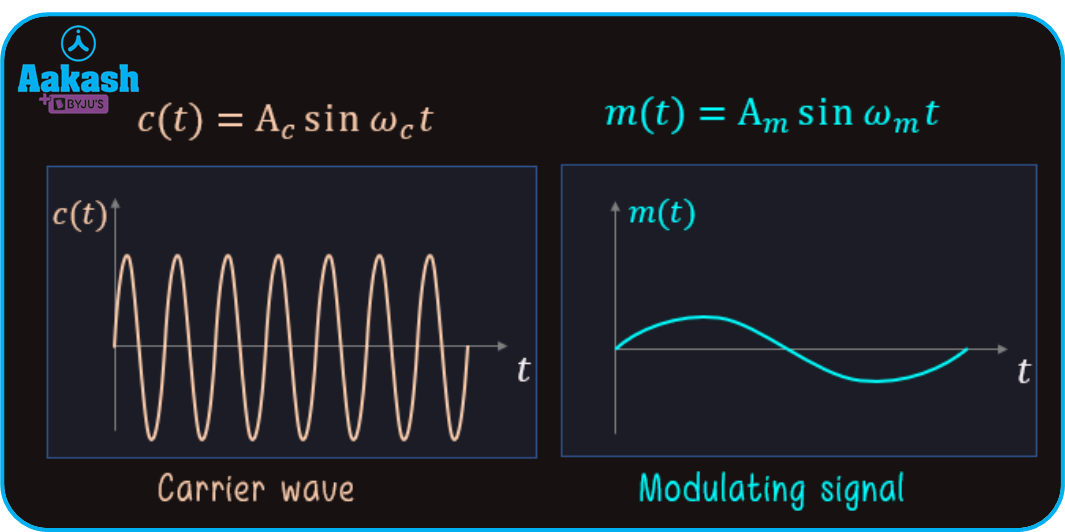Fig. shows the carrier wave and modulating wave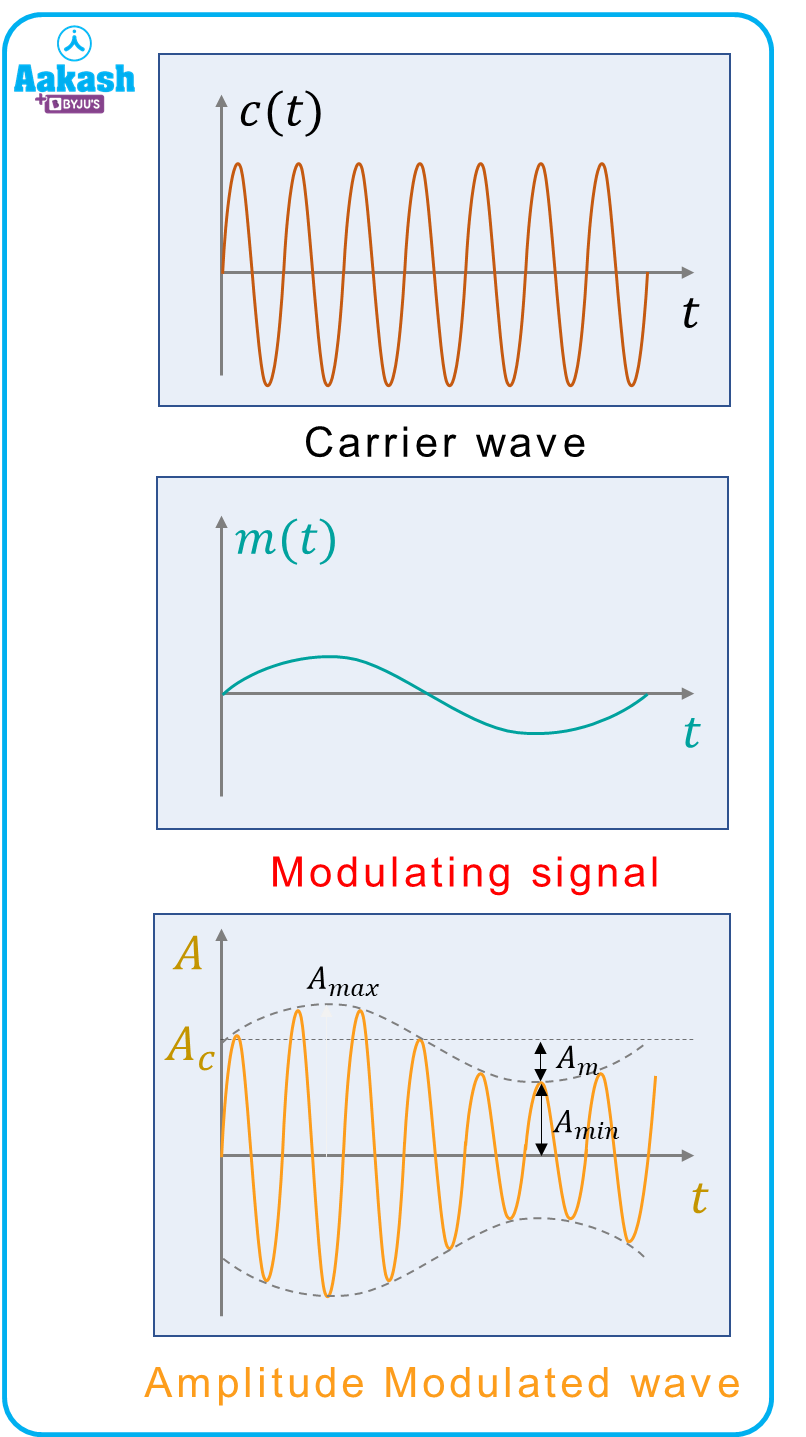Fig shows the amplitude modulated wave

From the above figure,

Modulation index / factor is given by,

Bandwidth of AM wave$={\omega }_{USB}-{\omega }_{LSB}$

$=\left({\omega }_{c}+{\omega }_{m}\right)-\left({\omega }_{c}-{\omega }_{m}\right)$

$=2{\omega }_{m}$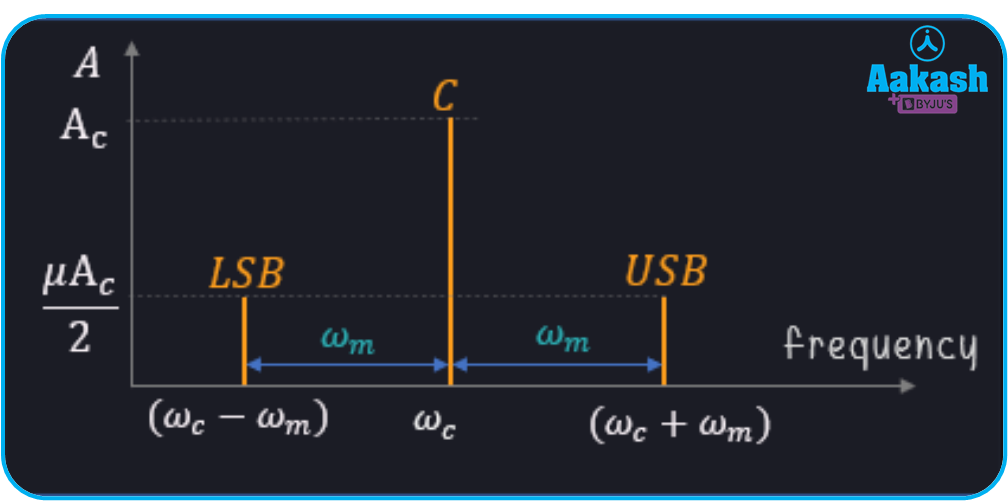Figure shows the bandwidth of AM wave

1. Frequency modulation: When a modulating audio frequency (AF) wave is superimposed on a high frequency carrier signal in such a way that the frequency of the carrier wave is kept different from the modulating signal, then the modulation is called frequency modulation.
1. Phase modulation: When a modulating audio frequency (AF) wave is superimposed on a high frequency carrier signal in such a way that the phase of the carrier wave is altered in accordance with the modulated signal, then the modulation is called phase modulation.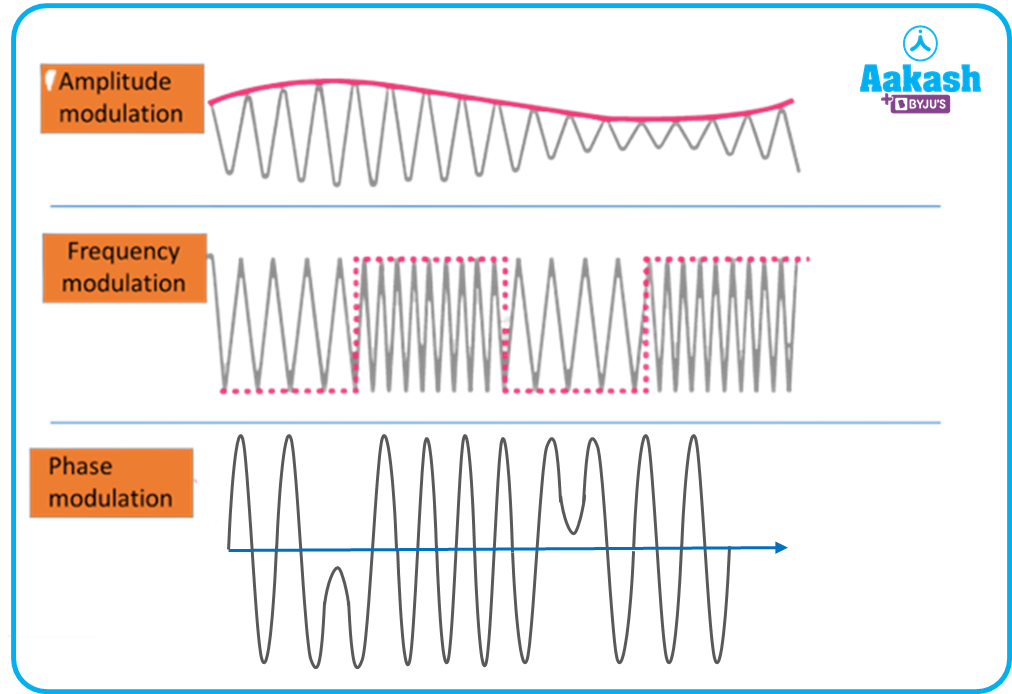Figure shows the AM waves, FM waves and PM waves

(b) Pulse wave modulation: The significant characteristics of a pulse can be varied in accordance with the modulating baseband (AF) signal, giving rise to;

1. Pulse amplitude modulation (PAM)
2. Pulse width modulation (PWM)
3. Pulse position modulation (PPM)

## Practice problems

Q. An audio signal having 10 kHz of frequency is to be sent to a receiver. Find out the height of the antenna. Also find out the possible height of antenna if the transmission frequency is increased to 2 MHz.

Ans: The frequency of the audio signal is, f=10 kHz

Then the corresponding wavelength is given by,

$\lambda =\frac{c}{f}$

Where, c= Speed of wave and f= audio signal frequency.

Then, the wavelength of the signal be,

Now we know the minimum length of an antenna should be /4. Then,

Hence the height of the antenna for the given frequency is 7500 m which is practically impossible to construct.

Now make a practically possible antenna, the transmission frequency is increased to 2 MHz. Then, the corresponding wavelength is given by,

Now the minimum height of the antenna will be

Hence the practically possible height of the antenna will be 37.50 m.

Q. If the carrier signal and modulating signal is represented by,

c(t)=505t V and m(t)=202t V

Then find the maximum and minimum amplitudes of an amplitude modulation wave.

Ans: The carrier signal and modulating signal is represented by,

Comparing above equation with

We get, Ac=50 V and Am=20 V

Then the maximum and minimum amplitudes of an amplitude modulation wave is given by,

Amax=Ac+Am=50+20=70 V

Amax=Ac-Am=50-20=30 V

Q. If the carrier signal and modulating signal is represented by,

c(t)=505t V and m(t)=202t V

Then find the modulation index.

Ans: The carrier signal and modulating signal is represented by,

c(t)=505t V and m(t)=205t V

Comparing above equation with c(t)=Acct and m(t)=Ammt

We get, Ac=50 V and Am=20 V

Then the modulation index is given by,

$\mu =\frac{{A}_{m}}{{A}_{c}}=\frac{20}{50}=0.4$

## FAQs

Q. What is modulation?
A.
It is a process of transmission of an information signal to a receiver in which a high frequency signal, called a carrier signal, is superimposed over a low frequency information signal.

Q. Explain the difference between frequency modulation and amplitude modulation?
A.
In the amplitude modulation, the alteration is done in amplitudes of the carrier signal and the message signal. In the frequency modulation, the alteration is done in the frequencies of the carrier signal and the message signal.

Q. Write the condition for efficient transmission, related to the power radiated from the antenna.
A.
Since the relation between effective power radiated for a given length of antenna is,

Where, l= length of the antenna and = wavelength of the transmitted signal

For a good transmission, high power is required for a given length of antenna and it is obtained by using low wavelength or high frequency for the transmission signal.

Q. Why is modulation required before the transmission of an information signal?
A.
Since the information signal is a very low frequency signal (about 20 Hz-20kHz) and can not be transmitted over long distances and also there are multiple numbers of information signals are transmitting continuously from the transmitter to receiver, so there might be chances of mixing of signals which will cause misinformation as a result. Therefore modulation is required before the transmission of the information signal. Since in the process of modulation, a high-frequency carrier signal carries the information signal, the height of the antenna will also be decreased.Talk to our expert
Resend OTP Timer =
By submitting up, I agree to receive all the Whatsapp communication on my registered number and Aakash terms and conditions and privacy policy